Question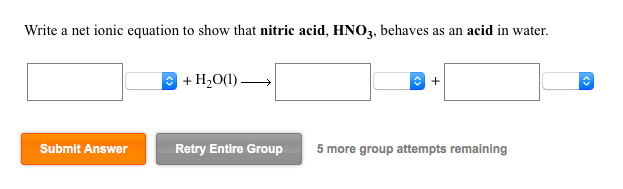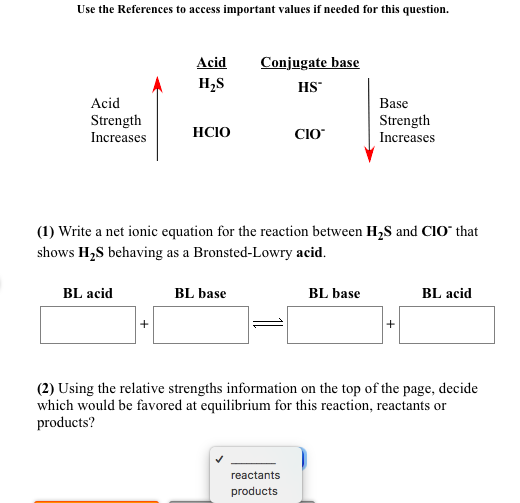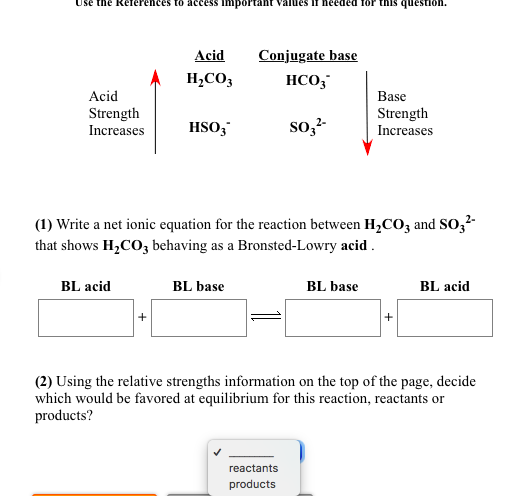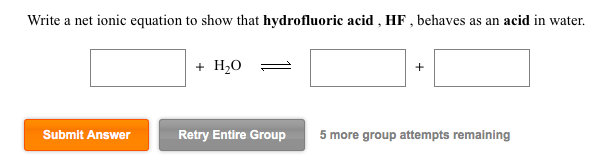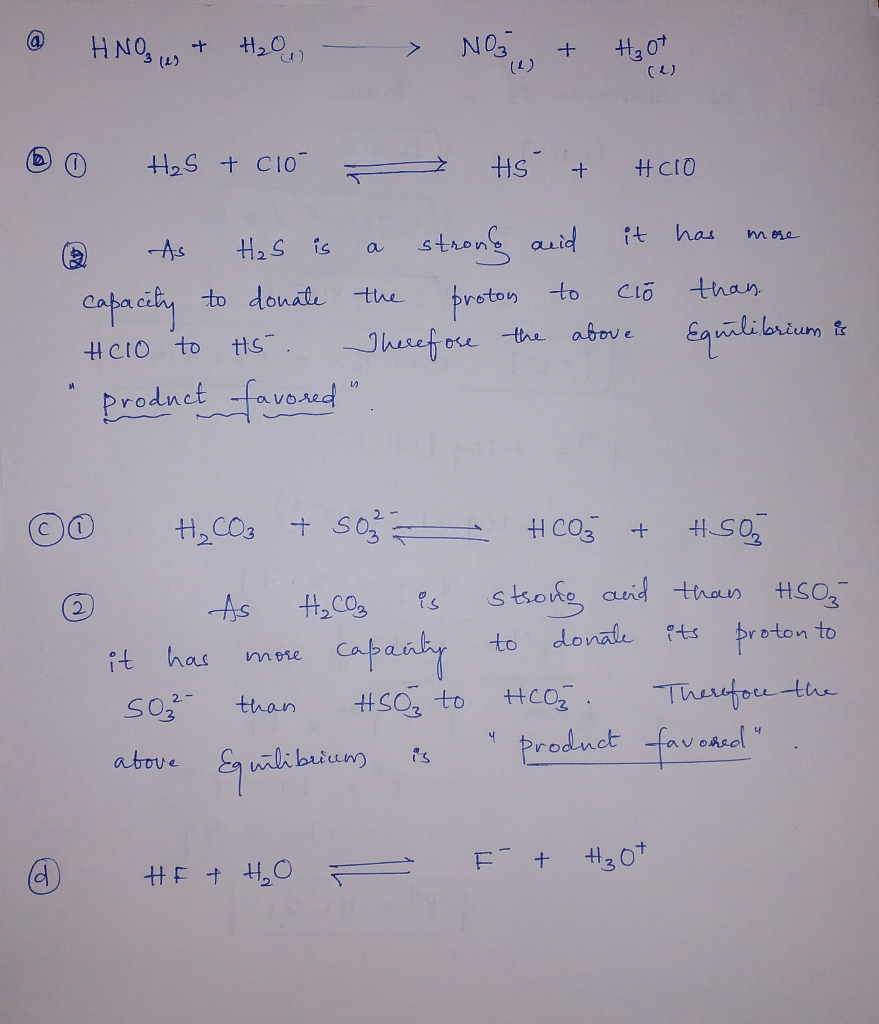Thank you vmuch....

#### Earn Coins

Coins can be redeemed for fabulous gifts.

Similar Homework Help Questions
• ### Write a net ionic equation to show that hydrocyanic acid behaves as a Brønsted-Lowry acid in...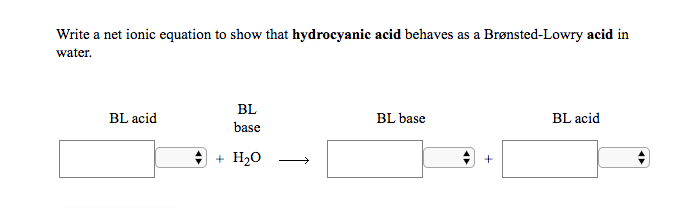Write a net ionic equation to show that hydrocyanic acid behaves as a Brønsted-Lowry acid in water. BL BL acid BL base BL acid base Write a net ionic equation to show that ethylamine, C2H5NH2, behaves as a Bronsted-Lowry base in water. BL BL base BL acid BL base acid + H20 Identify whether each species functions as a Brønsted-Lowry acid or a Brønsted-Lowry base in this net ionic equation. + CIO = HSO3 + HCIO H2SO3(aq) Brønsted-Lowry Brønsted-Lowry Brønsted-Lowry...

• ### Write a net ionic equation to show that methylamine, CH3NH2, behaves as a Bronsted-Lowry base in...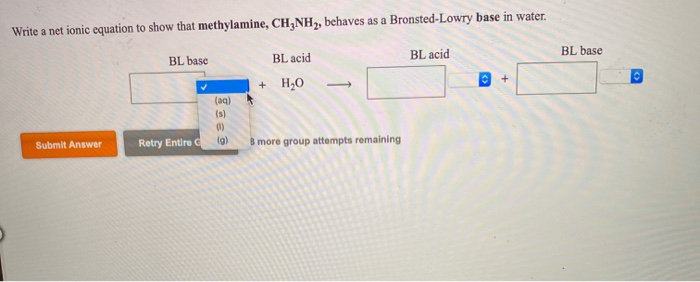Write a net ionic equation to show that methylamine, CH3NH2, behaves as a Bronsted-Lowry base in water. BL base BL acid BL base BL acid + H2O (aq) Submit Answer Retry Entire (g) B more group attempts remaining

• ### Write a net ionic equation to show that acetic acid behaves as a Brønsted-Lowry acid in...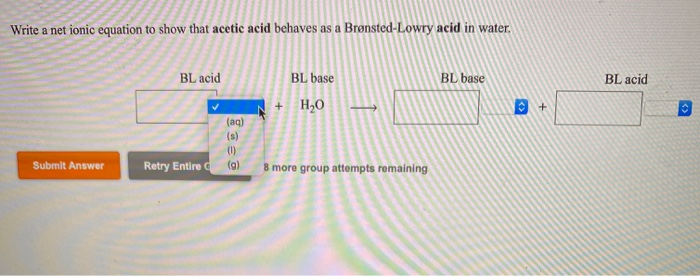Write a net ionic equation to show that acetic acid behaves as a Brønsted-Lowry acid in water. BLacid BL base BL acid C + Submit Answer Retry Entire (9) 8 more group attempts remaining

• ### (1) Write a net ionic equation for the reaction between HSO3 and CH3COOH that shows HSO;...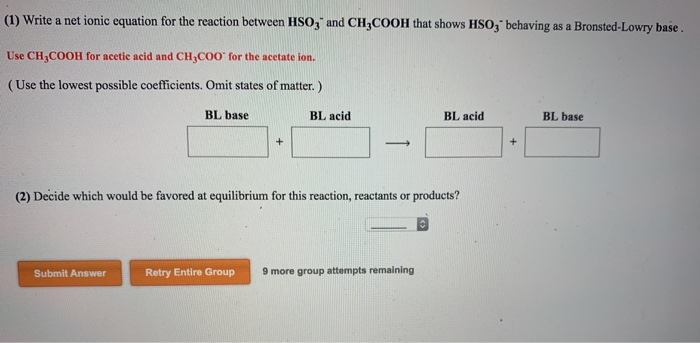(1) Write a net ionic equation for the reaction between HSO3 and CH3COOH that shows HSO; behaving as a Bronsted-Lowry base. Use CH3COOH for acetic acid and CH C00 for the acetate ion. (Use the lowest possible coefficients. Omit states of matter.) BL base BL acid BL acid BL base + + (2) Decide which would be favored at equilibrium for this reaction, reactants or products? Submit Answer Retry Entire Group 9 more group attempts remaining

• ### Use the References to access important values if needed for this question. (1) Write a net...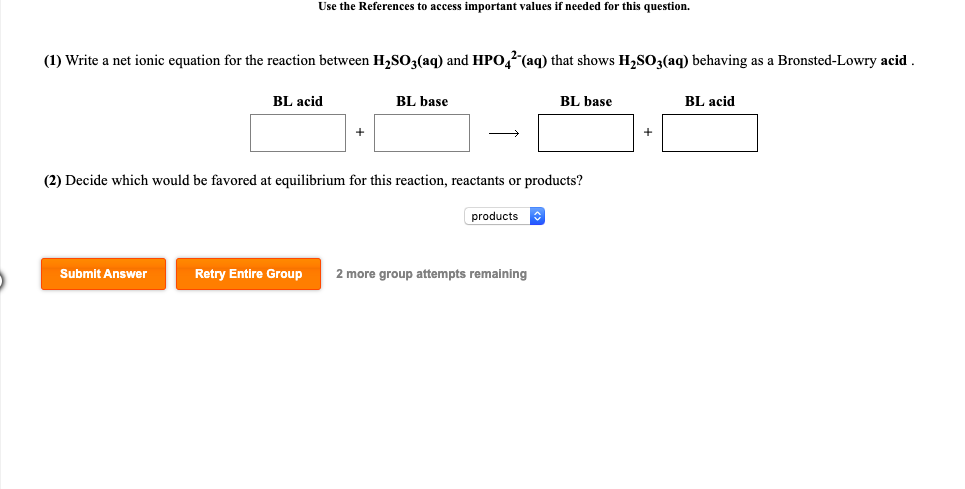Use the References to access important values if needed for this question. (1) Write a net ionic equation for the reaction between H2SO3(aq) and HPO (aq) that shows H2SO3(aq) behaving as a Bronsted-Lowry acid. BL acid BL base BL base BL acid (2) Decide which would be favored at equilibrium for this reaction, reactants or products? products Submit Answer Retry Entire Group 2 more group attempts remaining

• ### Write a net ionic equation to show that pyridine, C5H5N, behaves as a Bronsted-Lowry base in...

Write a net ionic equation to show that pyridine, C5H5N, behaves as a Bronsted-Lowry base in water. BL base BL acid BL acid BL base + H2O +

• ### Use the References to access important values if needed for this question. (1) Write a net...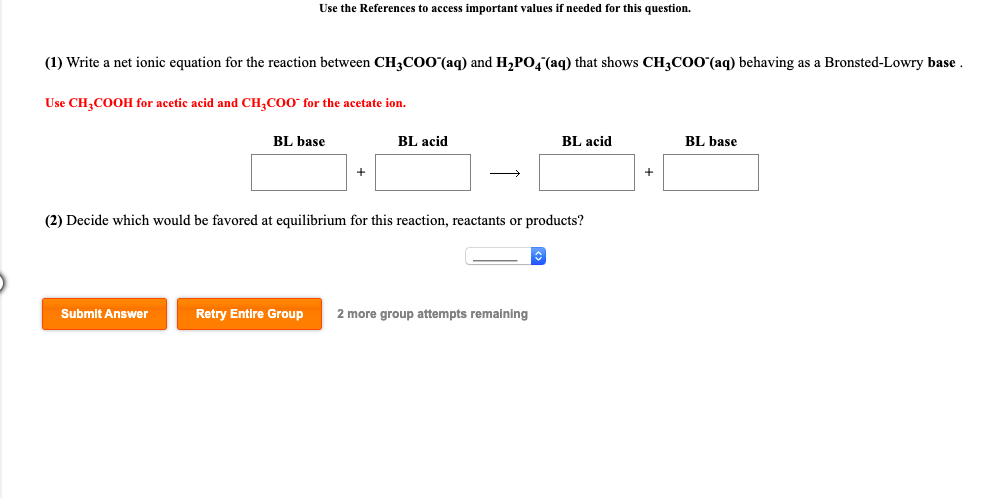Use the References to access important values if needed for this question. (1) Write a net ionic equation for the reaction between CH3C00"(aq) and H2PO4 (aq) that shows CH3C00"(aq) behaving as a Bronsted-Lowry base. Use CH COOH for acetic acid and CH COO for the acetate ion. BL base BL acid BL acid BL base (2) Decide which would be favored at equilibrium for this reaction, reactants or products? Submit Answer Retry Entire Group 2 more group attempts remaining

• ### Write a net ionic equation to show that codeine, C,H,O,N, behaves as a Bronsted-Lowry base in...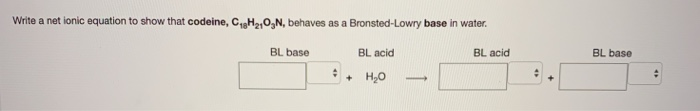Write a net ionic equation to show that codeine, C,H,O,N, behaves as a Bronsted-Lowry base in water. BL base BL acid BL acid BL base + H20 –

• ### Write a net ionic equation to show that formic acid, HCOOH, behaves as a Brønsted-Lowry acid...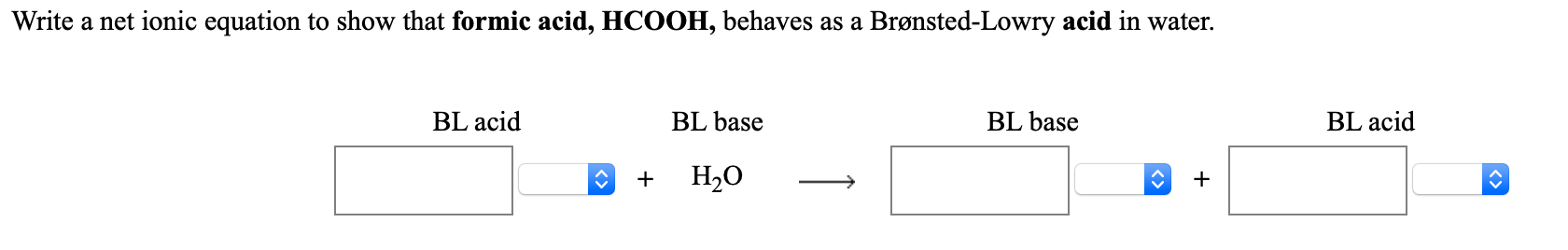Write a net ionic equation to show that formic acid, HCOOH, behaves as a Brønsted-Lowry acid in water. BL acid BL base BL base BL acid + H2O +

• ### [Review Toples) [References) Use the References to access important values if needed for this question. Write...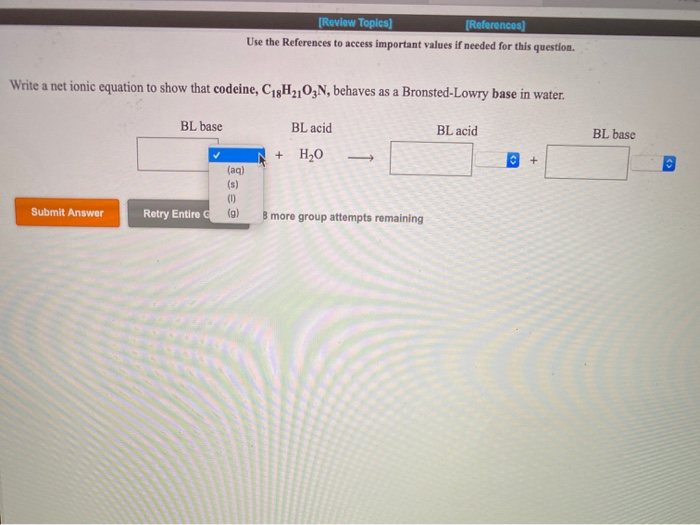[Review Toples) [References) Use the References to access important values if needed for this question. Write a net ionic equation to show that codeine, C18H2103N, behaves as a Bronsted-Lowry base in water. BL base BL acid BL base BL acid H20 + - (a) Submit Answer Retry Entire C (g) B more group attempts remaining